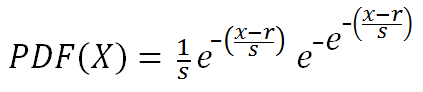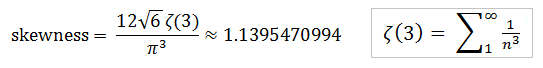# Gumbel Random Variable Generator

The Gumbel distribution with parameters r and s is often used to model the distribution of extreme values of natural phenomena. For instance, scientists may use it to predict earthquake magnitudes and flood levels. If you have many sets of random exponentially distributed random variables, the maximum values of the sets tend toward a Gumbel distribution.

Use the calculator below to generate random Gumbel variables for given r and s. The density function is discussed below.

 r =       s =       N = Decimal Precision integer tenths hundredths thousandths Display comma separated vertical

The Gumbel probability density function isThe mean, median, mode, and variance, of the Gumbel distribution depend on r and s:

mean = r + γs
median = r - ln(ln(2))s
mode = r
variance = (π²/6)s²

where γ is the Euler-Mascheroni constant 0.57721566... The skewness of the distribution is constant regardless of the parameter values. The exact value of the skewness is